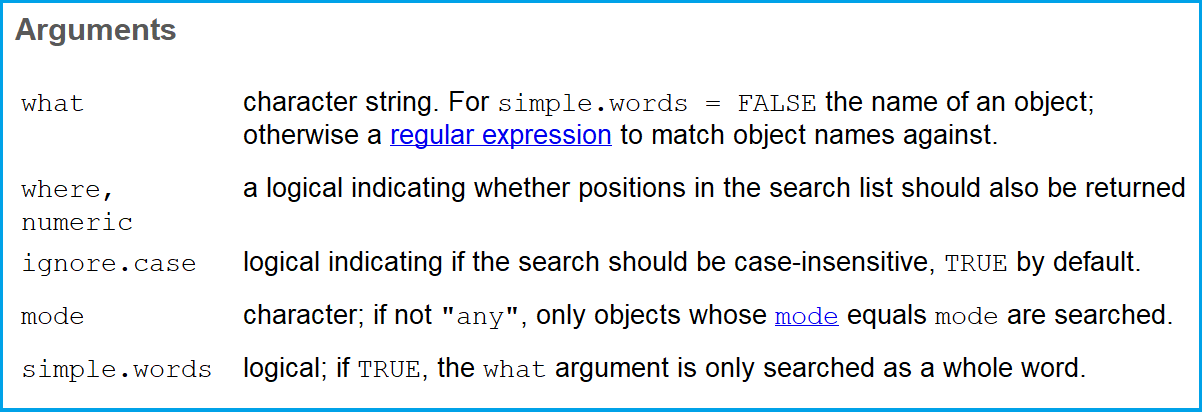# apropos & find Functions in R (2 Examples)

This tutorial explains how to find objects by (partial) names in the R programming language.

The tutorial will be based on the functions apropos() and find(). Let’s have a look at the basic R syntax and the definitions of the two functions:

Basic R Syntax of apropos & find:

```apropos("partial_name") find("name")```

Definitions of apropos & find:

The apropos R function returns a character vector giving the names of objects that contain the input character (partially).

The find R function returns the location where objects of a given name can be found.

In the following tutorial, I’ll show two examples for the application of apropos and find in R.

So now the part you have been waiting for…

## Example 1: apropos R Function

The apropos command is used to identify R objects that contain a character string at least partially.

Let’s see how this looks in practice:

```apropos("max") # Apply apropos function in R # "cummax" "max" "max.col" "pmax" "pmax.int" "promax" "varimax" "which.max"```

With the previous R code, we identified all R objects with a name containing the character “max”. Insert whatever character string you want in order to identify other R objects.

By the way: The apropos function allows for many different specifications and manual adjustments. You can find all options in the R help documentation:Figure 1: Arguments of apropos Function.

## Example 2: find R Function

The find command shows where an R object can be found. For instance, let’s find the location of the varimax function:

```find("varimax") # Apply find function in R # "package:stats"```

RStudio returns “package:stats”.

In other words: The varimax function is part of the stats R package.

## Further Resources for the Handling of Objects in R

Objects are an important concept of the R programming language. If you want to learn more about objects in R, you could have a look at the following YouTube video of Tutorlol:

Furthermore, you could check out the other R programming articles on my website. I’m publishing new R tutorials regularly:

In this article, I showed you how to identify and find objects in R. However, in case you have any further questions, don’t hesitate to let me know in the comments section below.